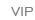# 橱柜衣柜

•[db:格式]

•[db:格式]

•[db:格式]

•[db:格式]

•[db:格式]

•[db:格式]

•[db:格式]

•[db:格式]

•[db:格式]

•[db:格式]

•[db:格式]

•[db:格式]

•[db:格式]

•[db:格式]

•[db:格式]

•[db:格式]

•[db:格式]

•[db:格式]

•[db:格式]

•[db:格式]

•[db:格式]

•[db:格式]

•[db:格式]

•[db:格式]

•[db:格式]

•[db:格式]

•[db:格式]

•[db:格式]

•[db:格式]

•[db:格式]

••